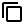# Add Fractions, Decimals, Integers and Mixed Numbers

## Add and Subtract Fractions, Mixed Fractions, Mixed Numbers, Add and Subtract Integers, Add and Subtract Decimals

HomeMath

You can input numbers, decimals, fractions, mixed fraction inside each box and get the total computed automatically.+

+

+

+

+

+

+

+

Remove

## How to add and subtract Whole numbers?• There are four Arithmetic operations. Addition, Subtraction, Multiplication and Division. In that the very basic one is addition.

Whole numbers are nothing but positive numbers with zero.

It is just like counting the numbers using your fingers

Adding two or more digit number is little different from adding single digit number.

• First align the numbers w.r.t their place values then add the digits in each place value.
Example: 28 + 61 :

H T O
2 8
+ 6 1
8 9

• Closure Property: Whole numbers are Closed(for ex: 4 + 9 = 13 4 and 9 the addend is a whole number and the result too is a whole number.)

• Commutative Property: If we change the order of the numbers the result won’t change.For eg: 4 + 5 = 9 = 5 + 4.

### How to add and subtract Integers?• Integers are set of positive numbers, negative numbers and zero with no decimal or fractional element.

#### We can perform following operations Addition of integers , subtraction of integers , Multiplication and Division of integers.

• Following are the example of integers: -42, -2 ,0 1,54,87

• But for adding negative numbers and combination of negative and positive numbers we have some rules to follow

• Here are the rules;

• When the two integers have the negative sign, add the integers and give (-) sign to the result.

• When the two integers have opposite sign [one positive (+) and other negative (-)], find the difference of the numbers and give bigger number sign to the result.

• Example: (-17) + 29

[Here, two integers are with unlike signs – and +.We find the difference of the numbers is 12 and to the result give greater number sign ; so the answer is positive 12]. = 12.

## How to add and subtract Decimals?• Decimal Addition is same as addition of whole numbers. Only thing we need to align the decimal numbers according to their place values one below the other, keeping the decimal point intact, and then add the numbers.

• Add zeros in the places wherever the length of the decimal numbers is not the same. Now add the numbers and place the decimal point aligned with the given decimal points.

• ### Adding decimals example: 2.53 + 1.14

O   t  h
2 .5 3
+1. 1  4
3. 6 7

• Then add the digits in each place value. Add the ones: 8+1=9 Add the tens: 2+6=8 , it is often easier to write the numbers vertically in columns.

• Begin to add decimals from the right-hand side,

• Decimal points are always aligned below the decimal point.

• Treat the decimal numbers as whole number and add at the end make sure to keep the decimal point in the proper place.• ### Closure Property of Addition:

Whole numbers, Natural, and Integers are Closed under addition.
For eg: 4 + 9 = 13 4 and 9 the addend is a whole number and the sum of the numbers too is a whole number.)

• ### Commutative Property of Addition:

If we change the order of the numbers the result won’t change.
For eg: 4 + 5 = 9 = 5 + 4.

• ### Inverse Property of Addition:

Inverse operation of addition is subtraction this property holds for whole , natural and Intergers.
For eg: 2 + 4 =6 and if we want to reverse the addition operation then subtract 4 from the result.This will give the original number with which we started.

• ### Associative Property of Addition:

Let a, b, and c be any three numbers.
a + (b + c) = (a + b) + c, (i.e) if we change the order of the numbers still the addition of numbers remains the same.
For eg: Lets take 3 , 4 , and 5. here 3 + (4 + 5) = 12 =(3 + 4) + 5

• ### Zero property of addition.

When we add the number to any of the number which will results in the same number.This number is known as Identity number.And this property is also known as Addition property of equality.
For eg: 4 + 0 = 4.

## Significant figures

• Definition: Significant figures of a given number is a number or digits that gives the accuracy of it.
We can perform significant figures addition.

• Rules to identify Significant figures

• Each and every non-zero numbers in a given number are significant.

• Zeros before the decimal are not significant.

• Zeros after the decimal are significant only if they are not followed by the non zero number.

• Zeroes between two non zero number are significant.

### Significant figure

#### 7 , 8 , 0 , 0